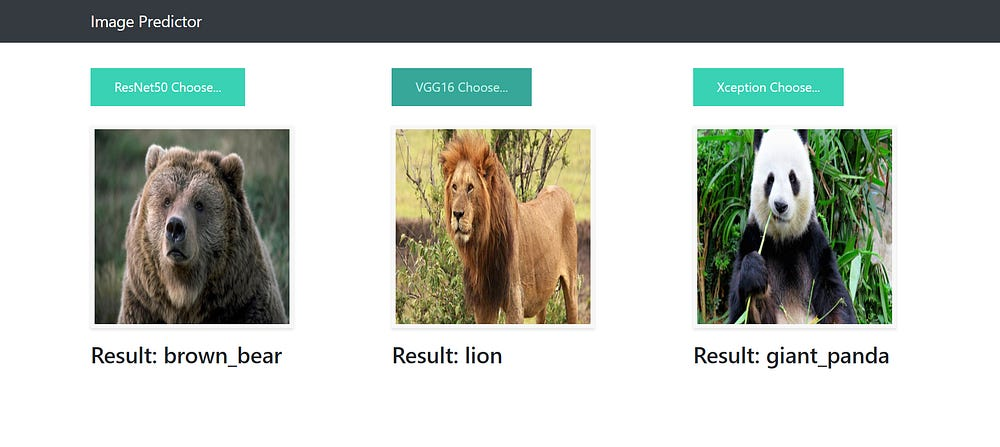# Deep Learning Keras and Flask as Web App

In this tutorial, we will present a simple method to take a Keras model and create Python Flask Web App.

Specifically, we will learn:

• How to load a Keras model into memory so it can be efficiently used for inference
• How to use the Flask web framework to create an endpoint for our API
• How to use the web api for our UI screen
• How to make predictions using our model, and return the results to the UI

### Configuring your development environment

We’ll be making the assumption that Keras is already configured and installed on your machine. If not, please ensure you install Keras using the official install instructions.

We’ll need to install Flask, it is a Python web framework, so we can build our API endpoint. We’ll also need requests so we can consume our API as well.

`\$ pip install flask gevent requests pillow`

Also we will use the requirements file. We use it to simple load dependencies.

Below is our requirements.txt file

`WerkzeugFlasknumpyKerasgeventpillowh5pytensorflow`

We must to use the below command to load dependencies

`\$ pip install -r requirements.txt`

Make sure you have the following installed:

• tensorflow
• keras
• pillow
• h5py
• gevent

### Create your Keras Rest Api

We create a app.py class and we use three image model (ResNet50, VGG16, Xception)

We include this libraries below.

`# ResNet50from keras.applications.resnet50 import ResNet50from keras.applications.imagenet_utils import preprocess_input as preprocess_input_resNet50, decode_predictions as decode_predictions_resNet50# VGG16from keras.applications.vgg16 import VGG16from keras.applications.vgg16 import preprocess_input as preprocess_input_vgg16, decode_predictions as decode_predictions_vgg16# Xceptionfrom keras.applications.xception import Xceptionfrom keras.applications.xception import preprocess_input as preprocess_input_xception, decode_predictions as decode_predictions_xception`

You can also use pretrained model from Keras Check https://keras.io/applications/

We will need to upload models that were created before

`modelResNet50 = ResNet50(weights='imagenet')print('ResNet50 Model loaded.')modelVGG16 = VGG16(weights='imagenet', include_top=True)print('VGG16 Model loaded.')modelXception = Xception(weights='imagenet', include_top=True)print('Xception Model loaded.')`

We will need to create API endpoint services

`@app.route('/', methods=['GET'])def index():    # Main page    return render_template('index.html')`

In the code above, it is redirected to the index.html page

`@app.route('/predictResNet50', methods=['GET', 'POST'])def predictResNet50():    if request.method == 'POST':        file_path = get_file_path_and_save(request)        img = image.load_img(file_path, target_size=(224, 224))        # Preprocessing the image        x = image.img_to_array(img)        # x = np.true_divide(x, 255)        x = np.expand_dims(x, axis=0)        # Be careful how your trained model deals with the input        # otherwise, it won't make correct prediction!        x = preprocess_input_resNet50(x, mode='caffe')        # Make prediction        preds = modelResNet50.predict(x)        # Process your result for human        # pred_class = preds.argmax(axis=-1)            # Simple argmax        pred_class = decode_predictions_resNet50(preds, top=1)   # ImageNet Decode        result = str(pred_class)               # Convert to string        return result    return None@app.route('/predictVGG16', methods=['GET', 'POST'])def predictVGG16():    if request.method == 'POST':        file_path = get_file_path_and_save(request)        img = image.load_img(file_path, target_size=(224, 224))        img_data = image.img_to_array(img)        img_data = np.expand_dims(img_data, axis=0)        img_data = preprocess_input_vgg16(img_data)        preds = modelVGG16.predict(img_data)        # decode the results into a list of tuples (class, description, probability)        pred_class = decode_predictions_vgg16(preds, top=1)        result = str(pred_class)  # Convert to string        return result    return None@app.route('/predictXception', methods=['GET', 'POST'])def predictXception():    if request.method == 'POST':        file_path = get_file_path_and_save(request)        img = image.load_img(file_path, target_size=(224, 224))        img_data = image.img_to_array(img)        img_data = np.expand_dims(img_data, axis=0)        img_data = preprocess_input_xception(img_data)        preds = modelXception.predict(img_data)        # decode the results into a list of tuples (class, description, probability)        pred_class = decode_predictions_xception(preds, top=1)        result = str(pred_class)  # Convert to string        return result    return None`

In the code above, we created three endpoint service . Their names are ResNet50, VGG16, Xception.

Firstly, We are recording the pictures from come to client. We are converting it to a matrix of 224 x 224. Then we are preprocess it.

Finally, we use a model to prediction and convert it string result. Than we send it back to the client.

### Starting the Keras Server

The Flask + Keras server can be started by running:

`\$ python app.py`
`ResNet50 Model loaded.VGG16 Model loaded.Xception Model loaded.Running on http://localhost:5000`

You can now access the Prediction Flask WebApp via `http://127.0.0.1:5000`

We have successfully called the Keras REST API and obtained the model’s predictions via Python and We have achieved successful estimates :)

Congratulations!!! Your first deep learning model deployed. You will find this example at my GitHub repository.

Peace. Happy Coding.

Source: Deep Learning on Medium